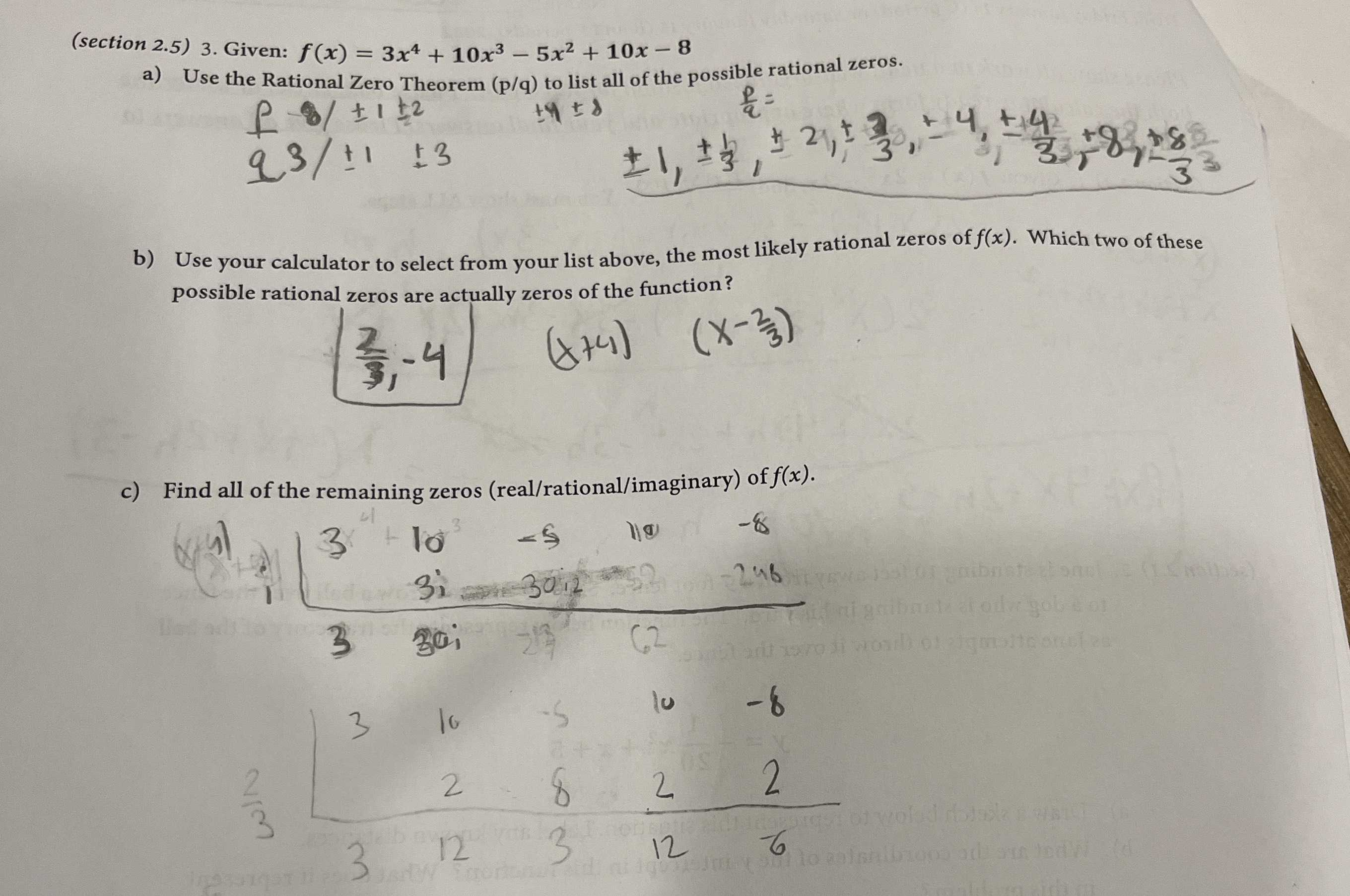### ¿Todavía tienes preguntas de matemáticas?

Pregunte a nuestros tutores expertos
Algebra
Pregunta3. Given: $$f ( x ) = 3 x ^ { 4 } + 10 x ^ { 3 } - 5 x ^ { 2 } + 10 x - 8$$

a) Use the Rational Zero Theorem ( $$/ 4 / q$$ ) to list all of the possible rational zeros.

b) Use your calculator to select from your list above, the most likely rational zeros of $$f ( x )$$ . Which two of these possible rational zeros are actually zeros of the function?

c) Find all of the remaining zeros (real/rational/imaginary) of $$f ( x )$$ .